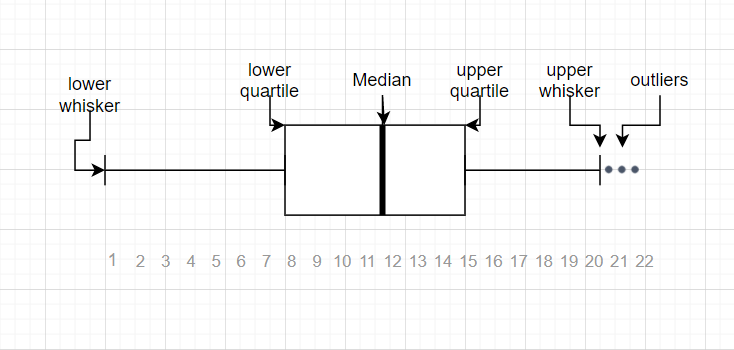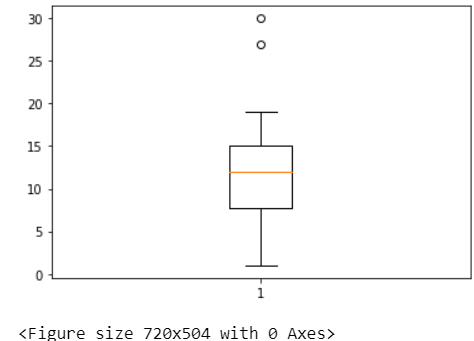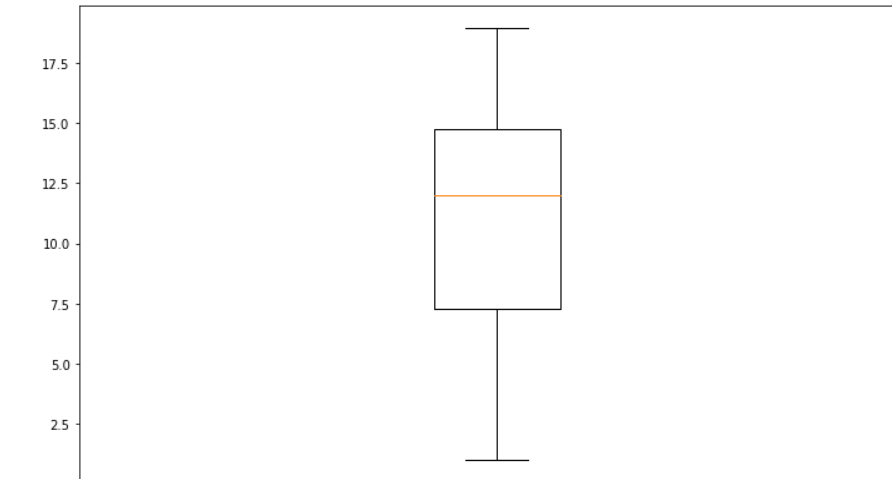Open in App
Not now

# Finding the outlier points from Matplotlib

• Difficulty Level : Basic
• Last Updated : 28 Jan, 2021

Outliers are the data points that differ from other observations or those which lie at a distance from the other data. They are mainly generated due to some experimental error which may cause several problems in statistical analysis. While in a big dataset it is quite obvious that some data will be further from the sample mean. These outliers need to be found and handle wisely.

We can use boxplots for the necessary.Above is a diagram of boxplot created to display the summary of data values along with its median, first quartile, third quartile, minimum and maximum. And the data points out of the lower and upper whiskers are outliers. In between the first and third quartile of whisker lies the interquartile region above which a vertical line passes known as the median. For further details refer to the blog Box plot using python. Following are the methods to find outliers from a boxplot :

```1.Visualizing through matplotlib boxplot using plt.boxplot().
2.Using 1.5 IQR rule.```

Example:

## Python3

 `# Adding libraries``import` `numpy as np``import` `pandas as pd``import` `matplotlib.pyplot as plt` `# random integers between 1 to 20``arr ``=` `np.random.randint(``1``, ``20``, size``=``30``)` `# two outliers taken``arr1 ``=` `np.append(arr, [``27``, ``30``])` `print``(``'Thus the array becomes{}'``.``format``(arr1))`

Output:

array([4, 12, 15,  7, 13,  2, 12, 11, 10, 12, 15,  5,  9, 16, 17,  2, 10, 15, 4, 16, 14, 19, 12,  8, 13,  3, 16, 10,  1, 13, 27, 30])

Visualizing by matplotlib boxplot using plt.boxplot()

## Python3

 `plt.boxplot(arr1)``fig ``=` `plt.figure(figsize ``=``(``10``, ``7``))``plt.show()`

Output:So from the above figure, we can witness the two outliers.

1.5 IQR Rule

Steps in 1.5IQR rule:-

• Finding the median, quartile, and interquartile regions
• Calculate 1.5*IQR below the first quartile and check for low outliers.
• Calculate 1.5*IQR above the third quartile and check for outliers.

## Python

 `# finding the 1st quartile``q1 ``=` `np.quantile(arr1, ``0.25``)` `# finding the 3rd quartile``q3 ``=` `np.quantile(arr1, ``0.75``)``med ``=` `np.median(arr1)` `# finding the iqr region``iqr ``=` `q3``-``q1` `# finding upper and lower whiskers``upper_bound ``=` `q3``+``(``1.5``*``iqr)``lower_bound ``=` `q1``-``(``1.5``*``iqr)``print``(iqr, upper_bound, lower_bound)`

Output:

`8.25 26.375 -6.625`

## Python3

 `outliers ``=` `arr1[(arr1 <``=` `lower_bound) | (arr1 >``=` `upper_bound)]``print``(``'The following are the outliers in the boxplot:{}'``.``format``(outliers))`

Output:

`The following are the outliers in the boxplot:[27 30]`

Thus, the outliers have been detected using the rule. Now eliminating them and plotting a graph with the data points-

## Python3

 `# boxplot of data within the whisker``arr2 ``=` `arr1[(arr1 >``=` `lower_bound) & (arr1 <``=` `upper_bound)]``plt.figure(figsize``=``(``12``, ``7``))``plt.boxplot(arr2)``plt.show()`

Output :My Personal Notes arrow_drop_up